# Samacheer Kalvi Books: Tamilnadu State Board Text Books Solutions

## Samacheer Kalvi 10th Maths Chapter 8 Statistics and Probability Notes PDF Download: Tamil Nadu STD 10th Maths Chapter 8 Statistics and Probability NotesSamacheer Kalvi 10th Maths Chapter 8 Statistics and Probability Notes PDF Download: Tamil Nadu STD 10th Maths Chapter 8 Statistics and Probability Notes

## Samacheer Kalvi 10th Maths Chapter 8 Statistics and Probability Notes PDF Download

We bring to you specially curated Samacheer Kalvi 10th Maths Chapter 8 Statistics and Probability Notes PDF which have been prepared by our subject experts after carefully following the trend of the exam in the last few years. The notes will not only serve for revision purposes, but also will have several cuts and easy methods to go about a difficult problem.

 Board Tamilnadu Board Study Material Notes Class Samacheer Kalvi 10th Maths Subject 10th Maths Chapter Chapter 8 Statistics and Probability Format PDF Provider Samacheer Kalvi Books

## How to Download Samacheer Kalvi 10th Maths Chapter 8 Statistics and Probability Notes PDFs?

2. Click on the Samacheer Kalvi 10th Maths Notes PDF.
3. Look for your preferred subject.
4. Now download the Samacheer Kalvi 10th Maths Chapter 8 Statistics and Probability notes PDF.

## Tamilnadu Samacheer Kalvi 10th Maths Solutions Chapter 8 Statistics and Probability Unit Exercise 8

Question 1.
The mean of the following frequency distribution is 62.8 and the sum of all frequencies is 50. Compute the missing frequencies f1 and f2.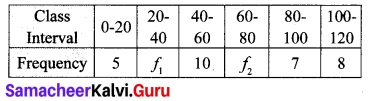Solution:
Mean x¯¯¯ = 62.8
Σx = 50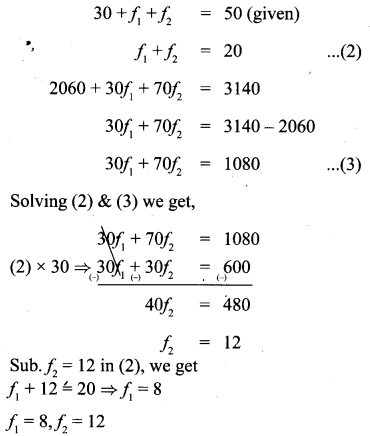Question 2.
The diameter of circles (in mm) drawn in a design are given below.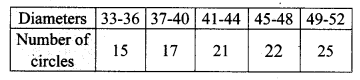Claculate the standard deviation.
Solution: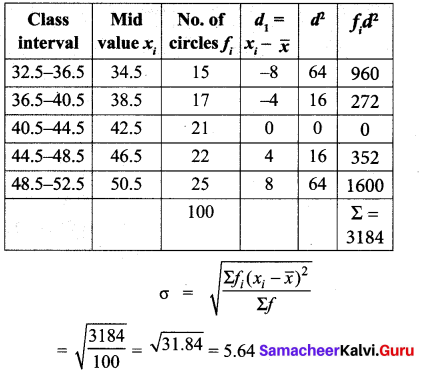Question 3.
The frequency distribution is given below.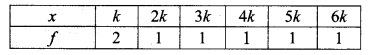In the table, k is a positive integer, has a varience of 160. Determine the value of k.
Solution: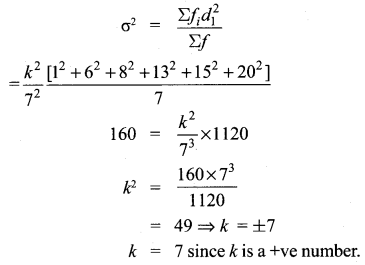Question 4.
The standard deviation of some temperature data in degree Celsius (°C) is 5. If the data were converted into degree Fahrenheit (°F) then what is the variance?
Standard deviation (σ) = 5
Variance = 52 = 25
We know the formula, F = 95 C + 32
Variance (F) = Vanance 95 C° + 32
[Variance of ax + b = a2 (variance of x)]
= (95)2 . variance
= 8125 × 25
= 81° F
New variance = 81° F

Question 5.
If for a distribution, Σ(x5)=3,Σ(x5)2=43 and total number of observations is 18, find the mean and standard deviation.
Solution: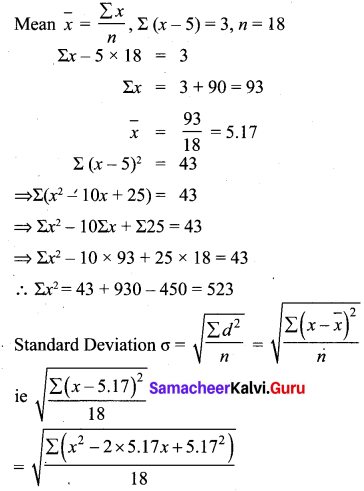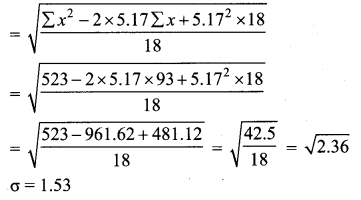Question 6.
Prices of peanut packets in various places of two cities are given below. In which city, prices were more stable?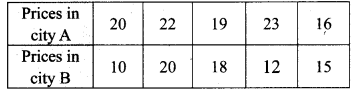Solution: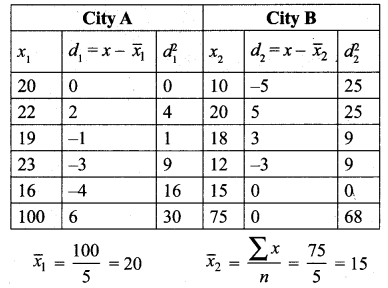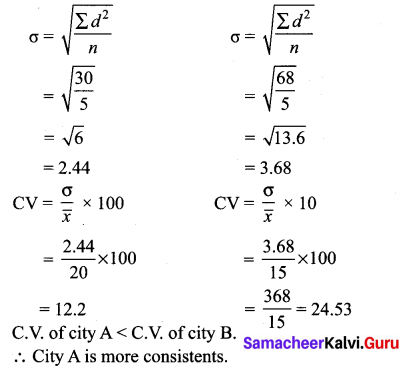Question 7.
If the range and coefficient of range of the data are 20 and 0.2 respectively, then find the largest and smallest values of the data.
Solution:
Range = L – S = 20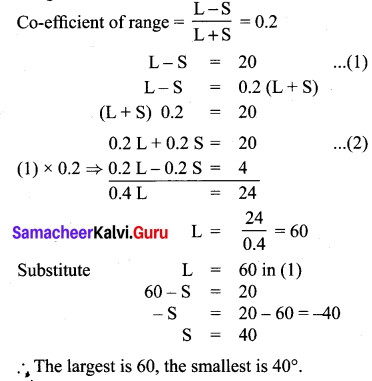Question 8.
If two dice are rolled, then find the probability of getting the product of face value 6 or the difference of face values 5.
Solution:
Product of face values 6: {(1, 6), (2, 3), (6, 1), (3,2)}
Difference of face value 5: {(1, 6), (6, 1)}Question 9.
In a two children family, find the probability that there is at least one girl in a family.
Solution:
S = {BB, BG, GB, GG}
n(S) = 4
Event of atleast one girl in a family say A
A= {BG, GB, GG}
n( A) = 3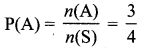Probability of at least one girl in a family is 34

Question 10.
A bag contains 5 white and some black balls. If the probability of drawing a black ball from the bag is twice the probability of drawing a white ball then find the number of black balls.
Solution:
Let a number of black balls be ‘x’.
Number of white balls = 5.Number of Black balls = 10.

Question 11.
The probability that a student will pass the final examination in both English and Tamil is 0.5 and the probability of passing neither is 0.1. If the probability of passing the English examination is 0.75, what is the probability of passing the Tamil examination?
Solution: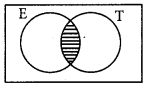P(English) = 0.75
P(Tamil) = x(assume)
P(English ∪ Tamil) = P(English) + P(Tamil) – P(English ∩ Tamil)
⇒ 1 – 0.1 = 0.75 + x – 0.5
⇒ x = 0.9 – 0.25Question 12.
The King, Queen and Jack of the suit spade are removed from a deck of 52 cards. One card is selected from the remaining cards. Find the probability of getting
(i) a diamond
(ii) a queen
(iv) a heart card bearing the number 5.
Solution:
∴ total number of cards = 52 – 3 = 49.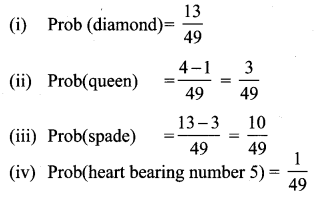## Tamilnadu Samacheer Kalvi 10th Maths Solutions Chapter 8 Statistics and Probability Additional Questions

Question 1.
Find the standard deviation of 30, 80, 60, 70, 20, 40, 50 using the direct method.
Solution:
Direct method: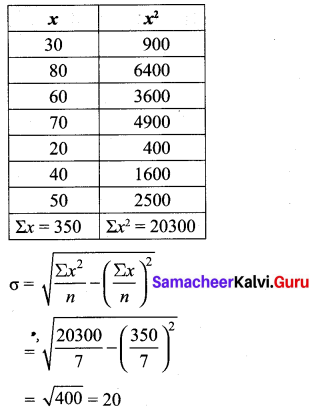Question 2.
Find the standard deviation for the following data. 5, 10, 15, 20, 25. And also find the new
S.D. if three is added to each value.
Solution: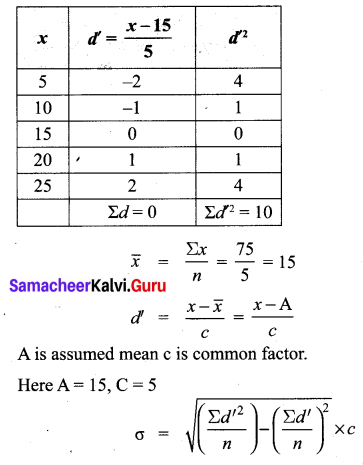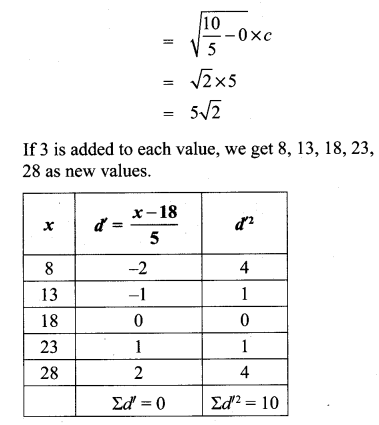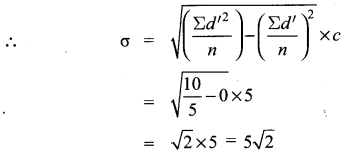S.D. doesn’t change when a number is added or subtracted to the values.

Question 3.
The marks scored by 5 students in a test for 50 marks are 20, 25, 30, 35, 40. Find the S.D for the marks. If the marks are converted for 100 marks, find the S.D. for newly obtained marks.
Solution:
Let assumed mean A = 30
C = 5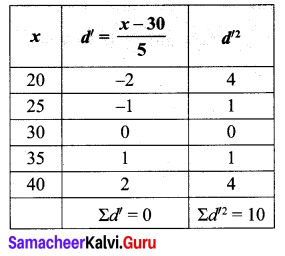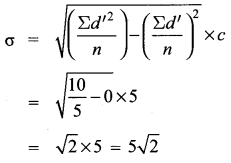To convert the values for 100, all the values will be multiplied by 2. Therefore the new values are 40, 50, 60, 70, 80.
Let A = 60,
C = 10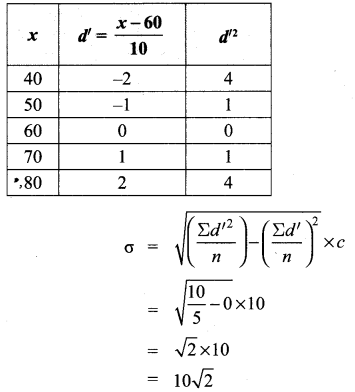S.D. also be multiplied by 2. It is also true for the division also.

Question 4.Solution: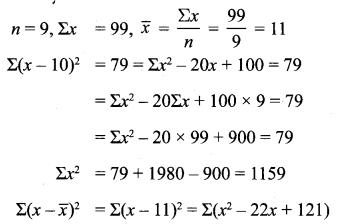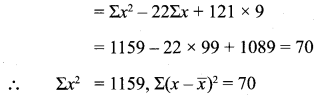Question 5.
Find the co-efficient of variation for the following data: 16, 13, 17, 21, 18.
Solution: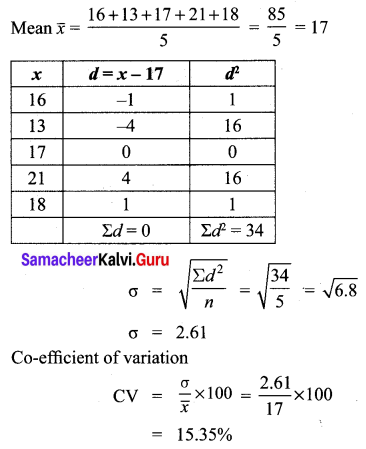Question 6.
C.V. of a data is 69%, S.D. is 15.6, then find its mean.
Solution: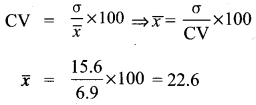Question 7.
S.D. of a data is 2102, mean is 36.6, then find its C.V.
Solution:Question 8.Which team is more consistent?
Solution: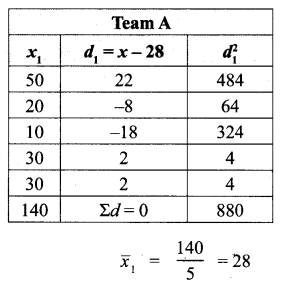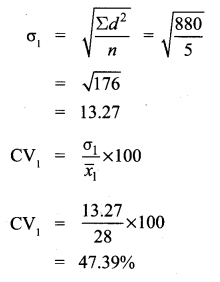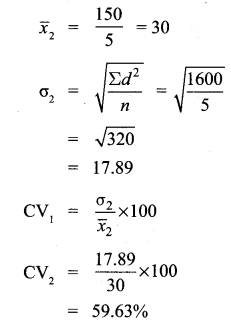∴ Team A is more consistent.

Question 9.
Final the probability of choosing a spade or a heart card from a deck of cards.
Solution:
Total number of cards = 52
Event of selecting a spade card = A
Event of selecting a heart card = B
n(A) = 13,
n(B) = 13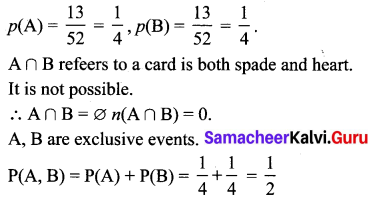## How to Prepare using Samacheer Kalvi 10th Maths Chapter 8 Statistics and Probability Notes PDF?

Students must prepare for the upcoming exams from Samacheer Kalvi 10th Maths Chapter 8 Statistics and Probability Notes PDF by following certain essential steps which are provided below.

• Use Samacheer Kalvi 10th Maths Chapter 8 Statistics and Probability notes by paying attention to facts and ideas.
• Pay attention to the important topics
• Refer TN Board books as well as the books recommended.
• Correctly follow the notes to reduce the number of questions being answered in the exam incorrectly
• Highlight and explain the concepts in details.

## Frequently Asked Questions on Samacheer Kalvi 10th Maths Chapter 8 Statistics and Probability Notes

#### How to use Samacheer Kalvi 10th Maths Chapter 8 Statistics and Probability Notes for preparation??

Read TN Board thoroughly, make separate notes for points you forget, formulae, reactions, diagrams. Highlight important points in the book itself and make use of the space provided in the margin to jot down other important points on the same topic from different sources.

#### How to make notes for Samacheer Kalvi 10th Maths Chapter 8 Statistics and Probability exam?

Read from hand-made notes prepared after understanding concepts, refrain from replicating from the textbook. Use highlighters for important points. Revise from these notes regularly and formulate your own tricks, shortcuts and mnemonics, mappings etc.
Share: